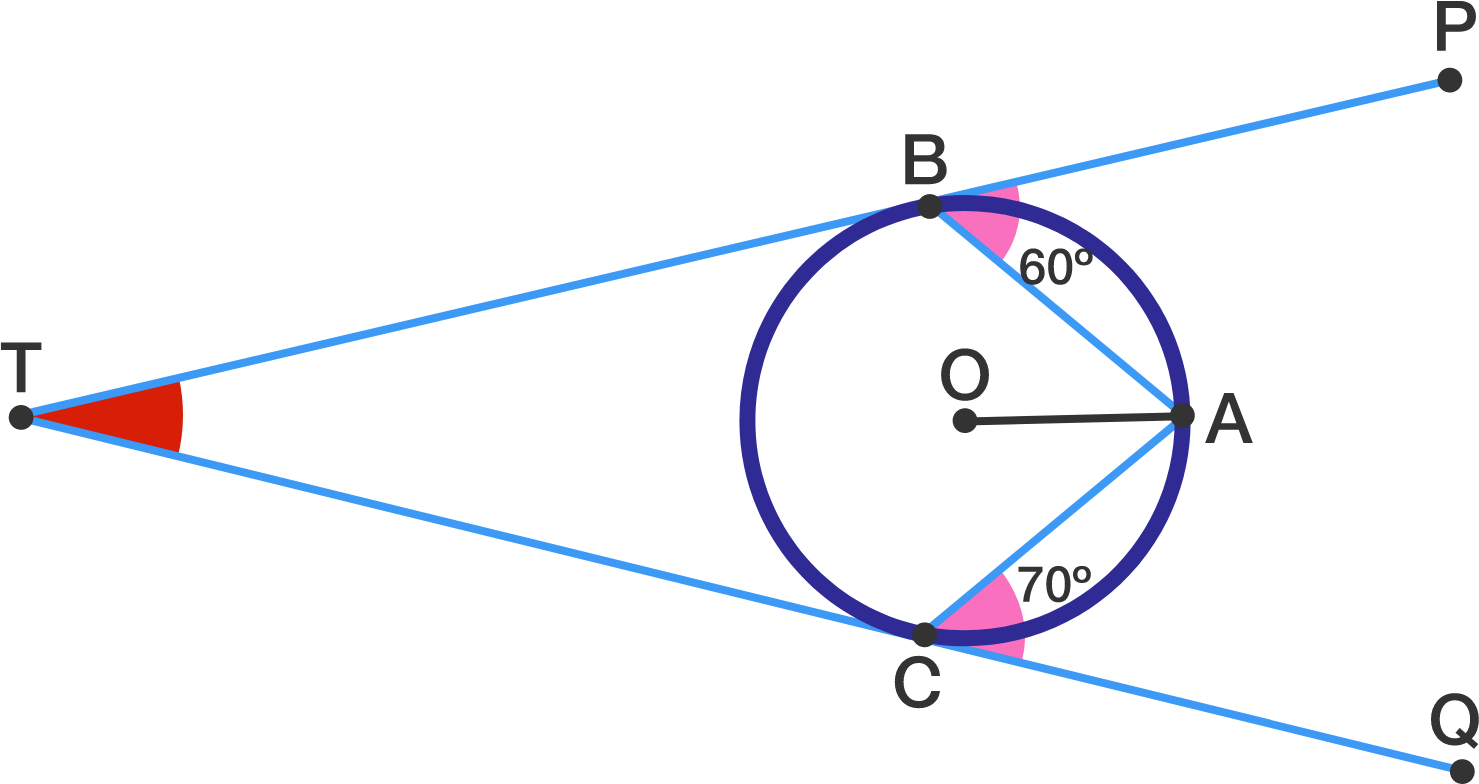# Circle Tangent Challenge

Geometry Level 2In the figure given above $\overline{TP}$ and $\overline{TQ}$ are tangent to the circle with center $O$ at $B$ and $C,$ respectively.

If $\angle PBA=60^\circ$and $\angle ACQ=70^\circ$, what is $\angle BTC$ in degrees?

×